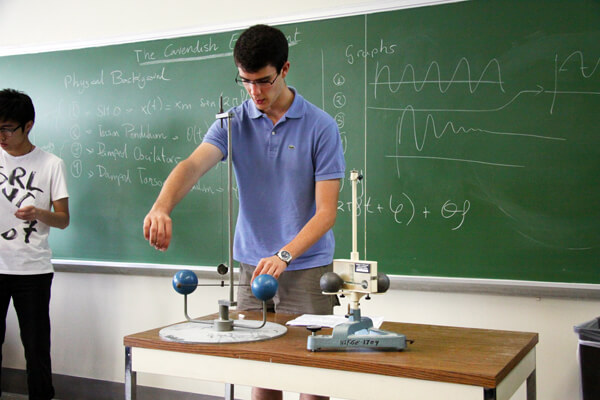## A Formula for Success in Physics: More Letters, Fewer Numbers.

One of the worst habits a student can develop in his first year of physics is to fill his worksheet with line after line of numbers. I think this approach is a big mistake; it may work for the simple problems one sees in the first few weeks or months of physics, but when the questions start to get complicated, it won’t work anymore. Worse, it’s unreliable, and leads to errors that are nearly impossible to detect. I’ve tutored many physics students over the years, and the first adjustment I’ve made with each one is to convince him of a simple principle: There should be only one line of math with real numbers in the solution to any physics problem. The rest should all be algebra. This approach helps minimize mistakes, prepare students to deal with more complex problems, and allow people to understand the physics of any problem instead of just getting lost in the maths.

Consider the following problem:

A 1 kilogram ball rolls at 5 meters/second off the edge of a 10 meter high roof. How fast is it going when it hits the ground? This is the sort of problem that you might see in your first week of an introductory physics course. The logic is pretty simple: How far does the ball move horizontally in the time it takes to fall to the ground? Once they understand how to think about this problem in two parts, most students will find the two necessary equations from a list and plug in the numbers given in the problem, separately. First, they will find the number of seconds it takes for the ball to hit the ground by plugging the given numbers into the equation h = ½*a*t2 and solving for t = 1.43 seconds. (h is the height of the roof, a is the acceleration due to gravity, and t is the time.) Then they will take this number and plug it into another equation for the horizontal motion, v = x/t, and solve for x = 7.14 meters.

So we have an answer: The ball will land 7.14 meters from the side of the building. But we have no way of making sense of this answer, no way of testing whether the system of equations we have set up accurately represents our physical intuition. This last part about intuition is the key. It’s the biggest reason why students who use too many numbers when solving problems feel lost when solving physics problems.

Instead of using numbers along the way, I suggest we algebraically solve the first equation for the time: t = √(h/[½*a]). Then, we can solve the second equation for the horizontal distance: x = v * t. Along the way, we can logically examine these equations to see if they agree with our physical intuition. For example, we know that if the roof gets higher (h increases), then the ball will fall for a longer time (i.e. it should increase). In the first of our two rearranged equations, h is on the top of the fraction inside the square root, so we know that as h increases, t will increase. Good, that matches our intuition. Similarly, in the second equation, we can see that as either the time (t) that the ball falls or the horizontal velocity (v) increases, the distance from the side of the building (x) should increase too. This also agrees with what we know from watching objects move around in the real world.

So now, we can combine the two equations, plugging the first rearranged equation into the second using t: x = v * √(h/[½*a]). Now we can analyze this equation in a similar way, checking that it matches our intuition: As height increases, x increases. As gravity gets stronger (a increases), x decreases. Both of these results make sense.

Now, we’re ready to use our one line of math with numbers. We plug in the values we know from the problem setup (h = 10 m, a = -9.8m/s2) and solve: x = 7.14 meters.

You might think these two methods are quite similar, and you’d be right. It’s easy enough to solve this beginner’s problem either way. But there are two reasons that adopting the algebraic method makes someone a stronger physics student:

1. The more often you use numbers to calculate, the more opportunities there are to make an avoidable mistake. This is especially true when questions try to trick you by introducing different units. We have no way of verifying that the intermediate results (e.g. the number of second, t = 1.43, we found using the first method) are correct, so we might be going down the wrong path. This problem gets much worse as the problems get more complicated.
2. Once we get our final answer, x = 7.14 m, there is no way to check whether that result reflects our physical intuition. At the end of solving the problem using the first method, there is no way to know how changing the height of the roof would vary the distance the ball travels. But when we wait to plug in numbers until the end, we can verify that increasing the height of the roof increases the distance the ball travels. When problems get more complicated, it is crucial to always check that your equations reflect the physics we understand intuitively.

When I start working with students, the first thing I always do is check how they use numbers and algebraic variables. Making this small change at the beginning of your physics career will pay huge dividends down the road. More letters, fewer numbers: That’s the formula for success in physics.